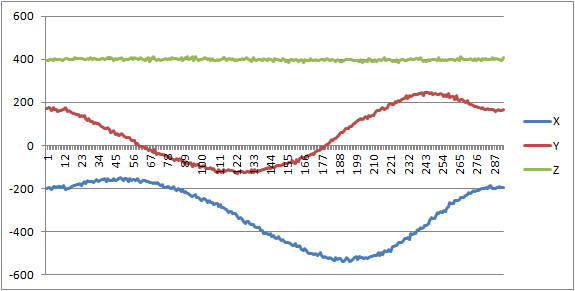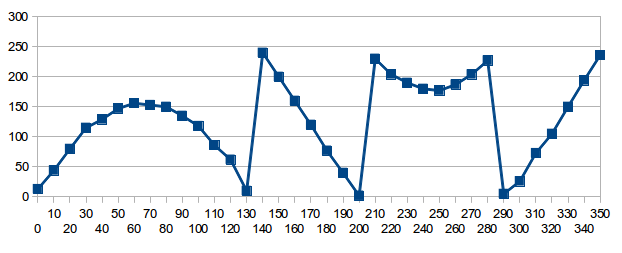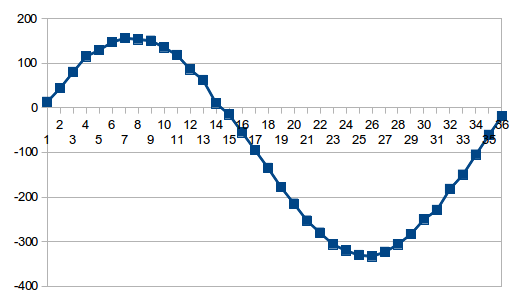Hello,
I rotate of magnetometer in a horizontal plane 360 degrees.
Raw data from magnetometer:

Convert data from two complement:

Сalculation Formulas (source):
Heading = arctan(Y/X) for X > 0 and Y >=0
= 180 + arctan (Y/X) for X < 0
= 360 + arctan (Y/X) for X > 0 and Y < 0
Result:

Where the error?

Hello, Jugin.

What are you using to communicate with the LSM303DLHC? Could you please post the code you are using and the raw data that you logged to make those graphs?

- Kevin

Hello, Kevin

I using OR-AVR-M128-S controller and OR-USB-UART. Programming language - jscript + hta.
LSM303DLHC сonnected by I2C.

Initialization:

1. Write address 0x3C register 0x02 value 0.
2. Verify - read from address 0x3d register 0x02 result - 0.

X = (Res << 8) | Res
Y = (Res << 8) | Res
Z = (Res << 8) | Res

If you have raw data from a magnetometer, maybe it would help me find the problem?

[quote=“Jugin”]X = (Res << 8) | Res
Y = (Res << 8) | Res
Z = (Res << 8) | Res[/quote]
Do Res through Res contain the values read from registers 03-08 in order? If so, you don’t seem to be combining them correctly. The order of the registers is:

03 OUT_X_H_M
04 OUT_X_L_M
05 OUT_Z_H_M
06 OUT_Z_L_M
07 OUT_Y_H_M
08 OUT_Y_L_M

Note that the high byte of each reading comes before the low byte, and Z comes before Y.

To help me better understand exactly what your code is doing, could you please show me your actual program in its entirety? You can upload it as an attachment or include it in your post and surround it in tags to make it easier to read. I do not have an LSM303DLHC set up to easily allow me to capture data for you, but if you can show me what the data you captured looks like, that would also be helpful. (I am specifically concerned that your graphs seem to show values in the ranges of 0 to 65535 or -32768 to 32767, since the readings from the magnetometer should only fall in the range of -2048 to 2047.)

- Kevin

The graph is exactly what you expect if the bytes are swapped. To check, I wrote a little program to generate the values i={-2048 to 2047}, incrementing by 4, then swapped them and displayed the result as an unsigned int k.

Here is a snippet of the output:

Hello,
Thank you very much, problem solved. Problem in “the bytes are swapped”. Sorry for my english.
After changing the order of bytes:After calibration X = X + 346, Y = Y - 58: (DeltaX = (min (X) + max(X))/2, DeltaY = (min (Y) + max(Y))/2)I attach an Excel document with the data, calculations and results.
Magnitometer.xls (149 KB)

I’m glad to hear that solved the problem. Thanks for the update.

- Kevin

Hello,

I have similar problem so I decided to write in this thread.
I have Attiny 2313 connected to LSM303DLHC thru I2C. I’ve connected everything and I can read data and pass it to eight digit LCD display. The problem is I’m receiving something like in the first post. The solution presented above (about swapping bytes) is not clear to me, so I’d appreciate any help with my code.

The code I’m using is from documentation - pages 10 and 11.
LSM303DLH-compass-app-note.pdf (767 KB)
I’m reading only high x,y,z bytes (because at that level I do not need great precision).

I’ve read the numbers from LCD and drew the chart like below (X had value 0,376,377 so I changed 376 to 276 to show the difference).

.

Code I’m, using is below

[code]include <avr/interrupt.h>
#define F_CPU 4000000UL
#include <stdlib.h>
#include <avr/io.h>
#include “i2cmaster.h”
#include <util/delay.h>
#include “lcd.h” /

#define KOMPAS 0x3C // address on i2c
#define CRA_REG_M 0x00 //from app-note
#define MR_REG_M 0x02 //from app-note

char MR_Data; //array to receive bytes
int Mx, My, Mz; //variables to maintain int values of bytes
unsigned char ret; //for connection
char buffer ; //for writing on lcd

int main(void)
{

``````lcd_init(LCD_DISP_ON);
i2c_init();
lcd_clrscr();

ret = i2c_start(KOMPAS+I2C_WRITE);
if ( ret ) {
lcd_puts("fail");
_delay_ms(5000);
}

i2c_write(CRA_REG_M);
i2c_write(0x14);
i2c_stop();

i2c_start_wait(KOMPAS+I2C_WRITE);
i2c_write(MR_REG_M);
i2c_write(0x0)
i2c_stop();
while (1)
{

i2c_start_wait(KOMPAS+I2C_WRITE);     // set device address and write mode
i2c_write(MR_REG_M);                        //
if ( ret ) {
lcd_puts("fail");
_delay_ms(5000);
}

i2c_stop();

//Mx = (int) (MR_Data << 8) + MR_Data;
//My = (int) (MR_Data << 8) + MR_Data;
//Mz = (int) (MR_Data << 8) + MR_Data;
``````

Mx = (int) MR_Data;
My = (int) MR_Data;
Mz = (int) MR_Data;

``````lcd_clrscr();
lcd_puts("x");
lcd_puts(itoa(Mx , buffer,8));
_delay_ms(500);
lcd_clrscr();
lcd_puts("y");
lcd_puts(itoa(My , buffer,8));
_delay_ms(500);
lcd_clrscr();
lcd_puts("z");
lcd_puts(itoa(Mz , buffer,8));
_delay_ms(500);
}
``````

}[/code]

Hello, rafar.

- Kevin

Thanks for help, but something is still wrong in my readings.
I added 10 as third argument to itoa and now X is constant zero, Y looks like my last chart, and Z is quite contant, but above zero (I’ll ignore it for now). Chart for Y is here:I’ve tried to change Y in libreoffice calc, so it’ll look like sinusoid and I achieved it by substracting 255 and 2*255 from values at certain points (numbers below), but I don’t understand the rule (below there are readings and my corrections), result here:Additionaly I tried to assign “-10” to my Mx value (Mx = (int) -10), and my LCD shows “-10” so itoa and my LCD can both use negative values, maybe the problem is with casting to int (Mx = (int) MR_Data;)?

```Y substracted Y substraction 13 13 44 44 80 80 115 115 129 129 147 147 156 156 153 153 150 150 135 135 118 118 86 86 62 62 10 10 240 -15 -255 200 -55 -255 160 -95 -255 120 -135 -255 77 -178 -255 40 -215 -255 2 -253 -255 230 -280 -510 204 -306 -510 190 -320 -510 180 -330 -510 177 -333 -510 187 -323 -510 204 -306 -510 227 -283 -510 5 -250 -255 26 -229 -255 73 -182 -255 105 -150 -255 150 -105 -255 194 -61 -255 236 -19 -255 ```

I noticed another problem with your original code (sorry I didn’t see it earlier). In this section, you are reading 6 registers, starting with MR_REG_M (because you write its register address in the second line):

``````   i2c_start_wait(KOMPAS+I2C_WRITE);     // set device address and write mode
i2c_write(MR_REG_M);                        //
if ( ret ) {
lcd_puts("fail");
_delay_ms(5000);
}